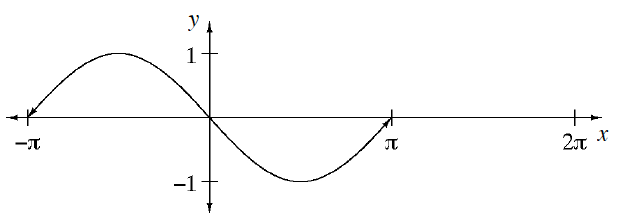### Home > INT3 > Chapter Ch9 > Lesson 9.2.1 > Problem9-99

9-99.The graph at right was made by shifting the first cycle of $y = \text{sin}\left(x\right)$ to the left. Homework Help ✎

1. How many units to the left was it shifted?

2. Figure out how to change the equation of $y = \text{sin}\left(x\right)$ so that the graph of the new equation will look like the one in part (a).

In the general equation $y = \text{sin}\left(x − h\right) + k$, which parameter moves the graph horizontally?